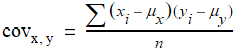# NMath User's Guide

38.9 Covariance, Correlation, and Autocorrelation (.NET, C#, CSharp, VB, Visual Basic, F#)

The static Covariance() method on class StatsFunctions computes the covariance of two data sets. Covariance is a measure of the tendency of two data sets to vary together, and is defined byEach deviation score in the first data set is multiplied by the corresponding deviation score in the second data set. For example:

Code Example – C#

```double cov = StatsFunctions.Covariance( data1, data2 );
```

Code Example – VB

```Dim Cov As Double = StatsFunctions.Covariance(MyData1, MyData2)
```

You can also specify a biased or unbiased estimator using values from the enumeration.

CovarianceMatrix() creates a square, symmetric matrix containing the variances and covariances of the columns in a given data matrix. The diagonal elements represent the variances for the columns; the off-diagonal elements represent the covariances of each pair of columns.

Correlation() calculates the correlation between two data sets. Correlation is covariance standardized by dividing by the standard deviation of each data set:The resultant value is the Pearson product-moment correlation coefficient, more commonly known simply as the correlation.

Spearmans() calculates the Spearman rank correlation coefficient, commonly known as Spearman's rho. Spearman's rho differs from Pearson's correlation only in that the computation is done after the values in the data set are converted to ranks (Section 38.5).

Fisher() calculates the Fisher transformation at a given value, which can be used to perform hypothesis testing on the correlation coefficient. FisherInv() calculates the inverse Fisher transformation.

Cronbach() calculates the standardized Cronbach's alpha test for reliability.

Autocorrelation is the correlation between members of a time series of observations. Class StatsFunctions provides two static methods for computing first-order autocorrelation:

DurbinWatson() calculates the Durbin-Watson statistic for the elements in a data set.

VonNeumannRatio() calculates the Von Neumann ratio for the elements in a data set.

For instance:

Code Example – C#

```double dw = StatsFunctions.DurbinWatson( data );
```
```double vnr = StatsFunctions.VonNeumannRatio( data );
```

Code Example – VB

```Dim DW As Double = StatsFunctions.DurbinWatson(MyData)
```
```Dim VNR As Double = StatsFunctions.VonNeumannRatio(MyData)
```

Top

Top